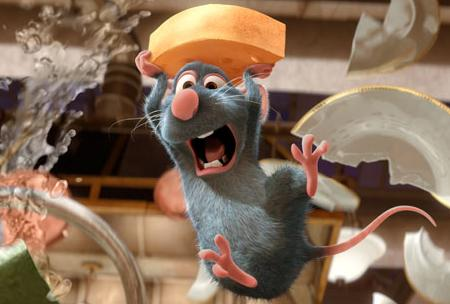Search IntMath
Close

# Math in Pixar animations

By Murray Bourne, 25 Mar 2008

Pixar is a well-regarded animation company, producing many hits like Toy Story, A Bug's Life, Finding Nemo and more recently, Ratatouille.

To produce a reasonable level of realism in these movies, you need to use sophisticated mathematics. Pixar does not animate by getting artists to draw every frame as was done in the early days of animation.

Everything is done using a computer, from lighting, movement, facial expressions, hair, collisions and 'tweening' (the process of producing frames that are in between key frames).

According to Pixar's How we Do It, each frame of the movie (1/24th of a second) takes about 6 hours to render, while some frames can take up to 90 hours. Rendering is the process where the computer puts together all the elements of a frame - color, lighting, outlines, textures, etc.Image source

There are some interesting short papers on the mathematics behind the animations here at Pixar's On-line Library. Many of the articles are about rendering and animation issues in creating Ratatouille and involve math topics like Bezier curves, RiCurve Geometry, Stochastic Simplification, vectors, biomechanics, Navier-Stokes equations and so on.

Applied math is alive and well - and vital - in computer-based animation.

Be the first to comment below.

### Comment Preview

HTML: You can use simple tags like <b>, <a href="...">, etc.

To enter math, you can can either:

1. Use simple calculator-like input in the following format (surround your math in backticks, or qq on tablet or phone):
a^2 = sqrt(b^2 + c^2)
(See more on ASCIIMath syntax); or
2. Use simple LaTeX in the following format. Surround your math with $$ and $$.
$$\int g dx = \sqrt{\frac{a}{b}}$$
(This is standard simple LaTeX.)

NOTE: You can mix both types of math entry in your comment.